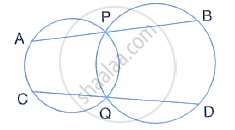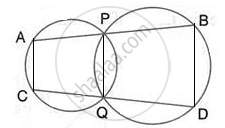Share

# Two Circle Intersect at P and Q. Through P, a Straight Line Apb is Drawn to Meet the Circles in a and B. Through Q, a Straight Line is Drawn to Meet the Circles at C and D. Prove that Ac is Parallel - Mathematics

Course

#### Question

Two circle intersect at P and Q. through P, a straight line APB is drawn to meet the circles in A
and B. Through Q, a straight line is drawn to meet the circles at C and D. prove that AC is
parallel to BD.#### SolutionJoin AC, PQ and BD
∴ ∠CAP + ∠PQC = 180°         ………….(i)
(pair of opposite in a cyclic quadrilateral are supplementary)
∴ ∠PQD + ∠DBP = 180°         ………….(ii)
(pair of opposite angles in a cyclic quadrilateral are supplementary)
Again, ∠PQC + ∠PQD = 180°      …………. (iii)
(CQD is a straight line)

Using (i), (ii) and (iii)
∴ ∠CAP + ∠DBP = 180° Or

∴ ∠CAB + ∠DBA =180°
We know, if a transversal intersects two lines such That a pair of interior angles on the same side of the Transversal is supplementary, then the two lines are parallel
∴  AC || BD

Is there an error in this question or solution?